# Balanced Forces

## Key Stage 3

### Meaning

Balanced Forces are forces that are the same strength but acting in opposite directions.

When forces are balanced an object will travel at a constant speed and in the same direction.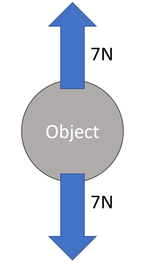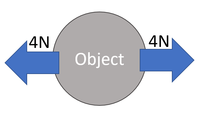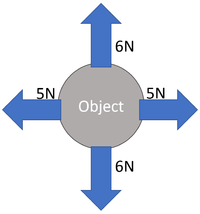The 7N up is balanced by the 7N down. The 4N left is balanced by the 4N right. The 6N up is balanced by the 6N down and the 5N left is balanced by the 5N right.

## Key Stage 4

### Meaning

Balanced forces are force vectors which add together to make 0N resultant force.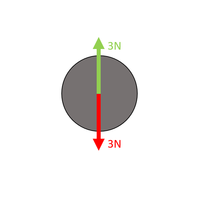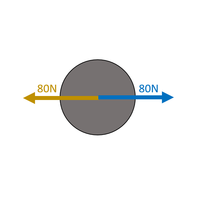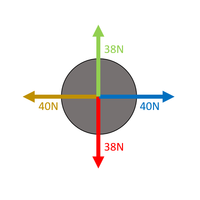$$F_R = F_{up} - F_{down}$$ $$F_R = 3 - 3$$ $$F_R = 0N$$ The forces are balanced. $$F_R = F_{right} - F_{left}$$ $$F_R = 80 - 80$$ $$F_R = 0N$$ The forces are balanced. $$F_{Horiztonal} = F_{right} - F_{left}$$ $$F_{Horiztonal} = 40 - 40$$ $$F_{Horiztonal} = 0N$$ $$F_{Vertical} = F_{up} - F_{down}$$ $$F_{Vertical} = 38 - 38$$ $$F_{Vertical} = 0N$$ The forces are balanced.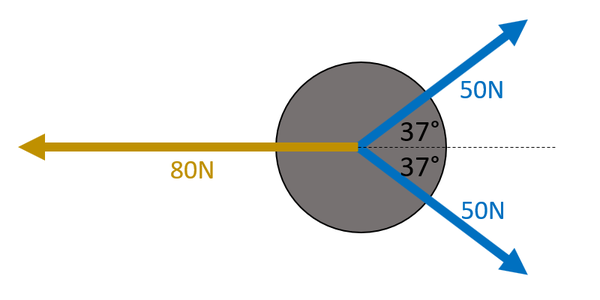Whether these forces are balanced can be found by resolving the blue force vectors into the horizontal. See Resolving Forces. $$F_{right} = 50\cos37 + 50\cos37$$ $$F_{right} = 79.86N$$ Since the angle in the question is given to two significant figures the answer should also be quoted to two significant figures. $$F_{right} \approx 80N$$ $$F_R = F_{right} - F_{left}$$ $$F_R = 80 - 80$$ $$F_R = 0N$$